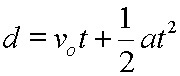## StatsMiniBlog: Odds and ProbabilitiesThere’s something that is frequently wittered about but the odds are you’ve never really been bothered enough to care if there’s a difference between ‘probability’ and ‘odds’ (like relative risk and odds ratios).
There are great reasons for this. Coffee, beer, ‘Take Me Out’ or a crash call to labour ward are four, for example. But given you’re here now … let’s address it.

Most stuff we naturally understand is probabilities; like 20%, 1 in 5, one fifth, or 0.2. They tell you the numbers with an event compared to the total number (n/N). It’s by comparing these measures of an event – which we tend, in lazy EBMspeak to call ‘risk’ – that we get ‘relative risks’ (RR)

20% risk of hypoglycaemia vs. 40% risk of  hypoglycaemia: RR = 20/10 = 2

Odds are different, though expressing the same idea. They tell you the numbers with an event, compared to the numbers without an event; 1:4, one quarter, 0.25. (They are n / {N-n} ) Comparing these gets you the odds ratio (OR):

Odds of 0.25 hypoglycaemia vs. Odds of 0.66 with hypoglycaemia: OR = 0.66/0.25 = 2.66

You’ll have probably noticed that

n/N
and
n/(N-n)

will be pretty much the same if n is quite small, in comparison to N but not if n is big … eg

1/2
and
1/(2-1) = 1/1

are quite different but

1/100
and
1/(100-1) = 1/99

are pretty much the same

Same is true for RR and OR. If the number of events cf the number of participants is small, then OR and RR are roughly equivalent. The closer you get to having evens and non-events being the same, the more different the two things become.

– Archi

(Visited 66 times, 1 visits today)